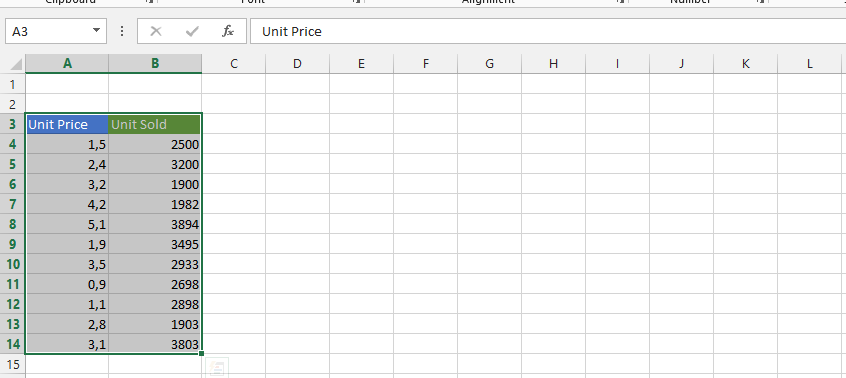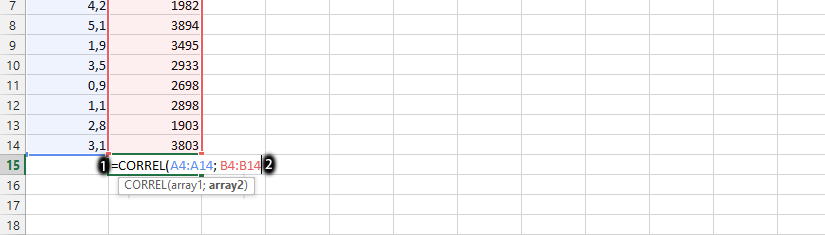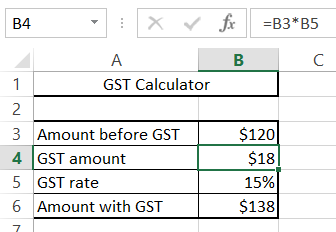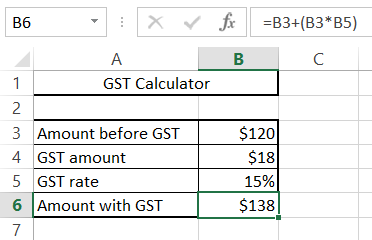#### How to calculate GST?

GST stands for Goods and Services Tax. GST is a value-added tax that is applied to the sale of goods and services in many countries, including India, Canada, and Australia. Calculating GST is going to be made easy, with these simple steps. We are going to calculate it together.

## Data preparation

What you’ll need:

• The GST rate for your country

Create a new Excel spreadsheet and enter the following columns:

• amount excluding GST tax
• the amount of gst
• gst rate
• amount including GST tax

In this Excel lesson, let’s create a GST calculator and calculate each of these values.

You should first layout the price before the GST.## Gst formula

The formula for calculating the gst tax is:

GST amount = amount before gst * gst rate

Click on the column beside GST amount, and decide the rate you would like the GST to be. We are going with 15%, and therefore writing = B3 * 0.15`Note: the GST amount has to be in form of percentage, and use only between 0.0 to 1.`

In my table, the amount before taxes is in cell B3. The tax rate is 15%. The formula is = B3 * 0.15, but you can also write it as = B3 * B5 because I entered the tax rate in cell B5.Calculations show that the gst amount is \$18.

## Calculation of the gst rate

In case you want to calculate the GST tax rate, you have two options.

### Calculation of the gst rate based on the price of the commodity

If you know the price of an item including tax and price excluding tax, use the following formula:

GST rate = (price with gst – price without gst) / price without gst

In my case, it will be the formula =(B6-B3)/B3

### Calculating the gst rate based on the tax amount and the price of the goods

If you know the amount of gst and the price of the goods excluding tax, then you should use the following formula:

GST rate = GST amount / price excluding GST

In the case I am discussing, you should use the formula =B4/B3

## Calculation of the price including gst

To calculate an amount that includes gst, you need an amount without gst. You also need to know the gst rate.

To calculate the price containing gst, use this formula:

Price including gst = price without gst + (price without gst * gst rate)

In the example below, the Excel formula is: =B3+(B3*B5). Use it to get the answer.## Calculation of the price without GST

To calculate a gst-free price, you need a gst-inclusive price. You also need to know the gst rate.

To calculate the price containing gst, use this formula:

Price without gst = price including gst / (1 + gst rate)

In the example below, the Excel formula is: =B6/(1+B5)

## Advanced GST Calculations in Excel

In this chapter, we will discuss some advanced GST calculations that you can do in Excel. These calculations will allow you to do more with your GST data, such as:

• Calculate GST for multiple countries
• Calculate GST for a range of prices
• Calculate GST for items that are exempt from GST
• Calculate GST for items that are subject to a reduced GST rate

### Calculating GST for multiple countries

If you are selling goods or services to customers in multiple countries, you will need to calculate GST for each country. You can do this by creating a separate Excel spreadsheet for each country and using the formulas in the previous chapter.

### Calculating GST for a range of prices

If you are selling goods or services at a variety of prices, you can use Excel to calculate GST for a range of prices. To do this, you can use the `VLOOKUP` function to look up the GST rate for a particular price.

### Calculating GST for items that are exempt from GST

Some items are exempt from GST. For example, food and medical supplies are often exempt from GST in many countries. You can use the `IF` function in Excel to check if an item is exempt from GST and then calculate GST accordingly.

### Calculating GST for items that are subject to a reduced GST rate

Some items are subject to a reduced GST rate. For example, books and newspapers are often subject to a reduced GST rate in many countries. You can use the `IF` function in Excel to check if an item is subject to a reduced GST rate and then calculate GST accordingly.

I hope this chapter has given you some additional insights into how to do advanced GST calculations in Excel. By following the techniques discussed in this chapter, you can improve your productivity and get more out of your GST data.

This is how to calculate GST in Excel. You can download free GST calculator template here